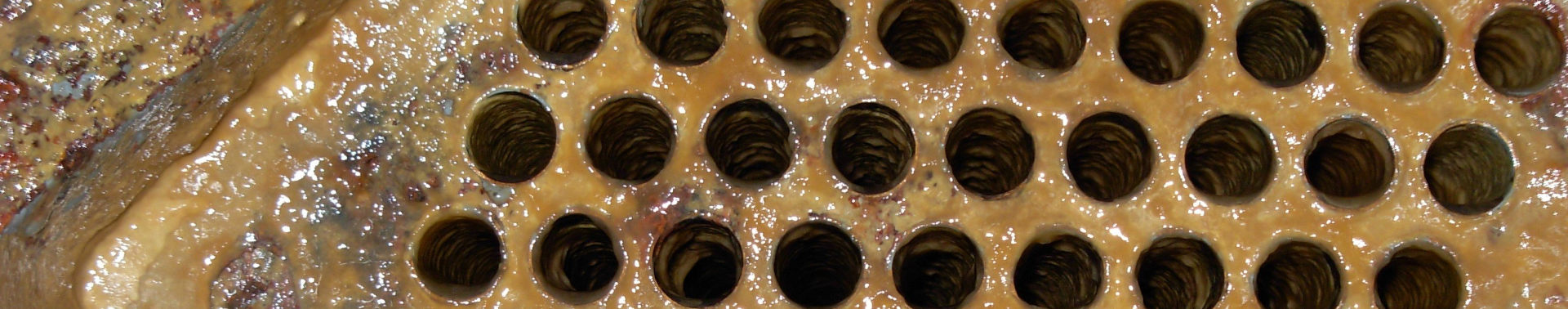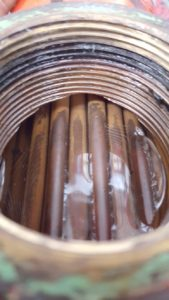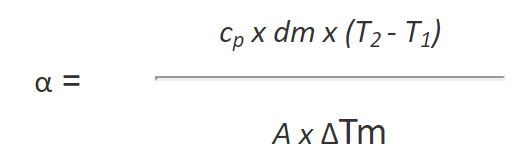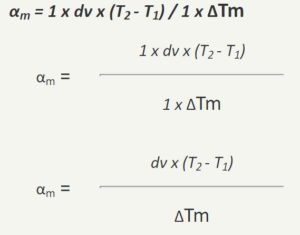﻿ Heat Transfer Coefficient used to the Heat Exchanger Performance# Heat Transfer Coefficient – Formula and CalculationThe heat transfer coefficient α is a calculated figure we can use to see indirect what is happening inside a heat exchanger. In an ideal world, the internal surface of a heat exchanger stays clean during its operation. If this is the case the heat transfer will stay constant as well, all good.

See the picture to the left. After installing Merus the tubes are clean, and there is a constant flow. In such clean tubes, or tubes with a very thin layer of incrustation, the heat transfer is very good.

As we are in most case not in an idle world, there happens fouling in heat exchangers. Means one can find all kind of incrustions. These incrustation are acting as an insulation. Less heat is transferred. We at Merus use the heat transfer to monitor the efficency of the Merus Rings.

## How to calculate the heat transfer coefficient

To calculate the coefficient α we need the relative easy accessible values of the temperature and the volume flow:

The transferred heat (Q) in a exchanger:
Q = α x A x (T2 - T1) x Δt
with: α = heat transfer coefficient, A = heat transfer surface, (T2 -T1)= temperature difference, Δt = examined time frame

The transferred heat (Q) of one of the liquids:
Q = cp x dm x (T2 - T1) x Δt
with: cp = heat capacity of the fluid, dm= mass flow of the fluid, (T2 - T1) = temperature difference, Δt = examined time frame

equalizing the both heat transfers as well as the average temperatures ΔTmresults in the heat transfer coefficient (α):For the control (development of heat transfer coefficient) there are no absolute values required, so it is possible to set A as constant (=1), and assuming always the same liquid cp and hence the same density ρ and set both as well as constant(=1). As the mass flow may be calculated with dm =  dv  x   ρ   (volume flow times density) we will get the "qualitative" Heat transfer coefficient αm .Using: T2, T1 the inlet and outlet temperature of the fluids and the volume flow. The heat loss is also taken as constant.

If you will need help, how to do the calculation or how to analyse / interpret your results from the field, please dont hesitate and write us an Email.

## The heat transfer coefficient is a process value

People are asking about the coefficient of water, or about the heat transfer of copper or steel. There is not such a thing, as the heat transfer is not depending only one material.
It is a process value of the heat transfer in between a fluid and a body.  For example from cooling water to the steel of a heat exchanger.

The heat transfer coefficient depends only on the fluid, its movement and the surface of the solid body. However, not from the material of the solid body.

To make it a little more clear:
Assumed we have tubes in water (e.g. tube bundles in a tubular heat exchanger). If the water stays still, the possible heat transfer is 350 to 500 W/(m2K), in case the water is running the possible heat transfer is 350 to 2100 W/(m2K). Having the same setup of the hardware and surroundings and change only the velocity of the water, one is able to transfer 4 times more energy. Guide values according to tables (Fig. VIII-5) [Kuchling].

People also calculate the U-value, which refers to the overall heat transfer coefficient. This is a more principal value, and gives at the heat exchanger design an indication how suitable certain materials are for constructing a heat transfer area.

So it can be understood how important it is to have clean surfaces. As dirt, limescale, rust or biomass all are lowering the heat transfer in a negative way.

We use cookies to ensure that we give you the best experience on our website and to improve the relevance of our communications with you. If you continue without changing your settings, we’ll assume that you are happy to receive all cookies on the merusonline website. However, if you would like to, you can change your cookie settings or find detailed information about how cookies are used on this website by going to 'Data protection'.